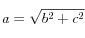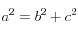Mathematical formulae

SPIP allows you to insert mathematical formulae using LaTeX notation, which will be automatically converted into an image inserted into the text.

You surround your mathematical "text" inside the $and$ tags. The LaTeX code for a formula will be placed between two "\$" dollar signs.

In this way, the text:

$the formula a = \sqrt{b^{2} + c^{2}} is a reformulation of Pythagorus' formula: a^{2} = b^{2} + c^{2}$

will be displayed as:

the formulais a reformulation of Pythagorus’ formula:An article in the documentation explains this functionality in greater depth.